# Simply-connected group

Jump to: navigation, search

A topological group (in particular, a Lie group) for which the underlying topological space is simply-connected. The significance of simply-connected groups in the theory of Lie groups is explained by the following theorems.

1) Every connected Lie group $G$ is isomorphic to the quotient group of a certain simply-connected group (called the universal covering of $G$) by a discrete central subgroup isomorphic to $\pi_1(G)$.

2) Two simply-connected Lie groups are isomorphic if and only if their Lie algebras are isomorphic; furthermore, every homomorphism of the Lie algebra of a simply-connected group $G_1$ into the Lie algebra of an arbitrary Lie group $G_2$ is the derivation of a (uniquely defined) homomorphism of $G_1$ into $G_2$.

The centre $Z$ of a simply-connected semi-simple compact or complex Lie group $G$ is finite. It is given in the following table for the various kinds of simple Lie groups.

<tbody> </tbody>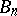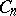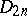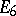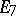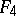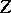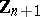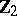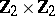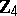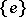In the theory of algebraic groups, a simply-connected group is a connected algebraic group $G$ not admitting any non-trivial isogeny $\phi : \tilde G \rightarrow G$, where $\tilde G$ is also a connected algebraic group. For semi-simple algebraic groups over the field of complex numbers this definition is equivalent to that given above.